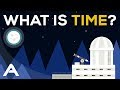What is Time ? - Encywiki

Trending

# What is Time ?

What is Time ?

What Is The Time

We can define time as a process of comparison of flows of two processes.
We compare the flow of one process with the progress of another one.
We may ask:"How often one process repeats while another one is still not ends.
To do the comparison we can use one process as standard(usually this is repeatable process) and compare with it the measured one.
As an analogy we can use the procedure of finding the length of the object.
We count how many times smaller object(measuring stick) "fits" in the measured one.
Then we "measure the time" we count how many times faster(shorter) process will "fits" in the bigger(longer) one.
Obviously, the shorter the standard process in accordance to the measured one - the more accurate result will be obtained.
Let's create hypothetical world. World where only Drop Clock in use.
What is the construction of this clock? Here it is.
In reservoir, filled with water, in the bottom, there is a small hole of such size, that water don't flows through this...
What is the time?, By Aleksandr Anufriyev
What is Time ?Reviewed by encywiki on April 13, 2019 Rating: 5 What is Time ? What Is The Time We can define time as a process of comparison of flows of two processes. We compare the flow...